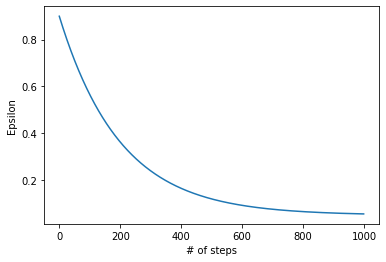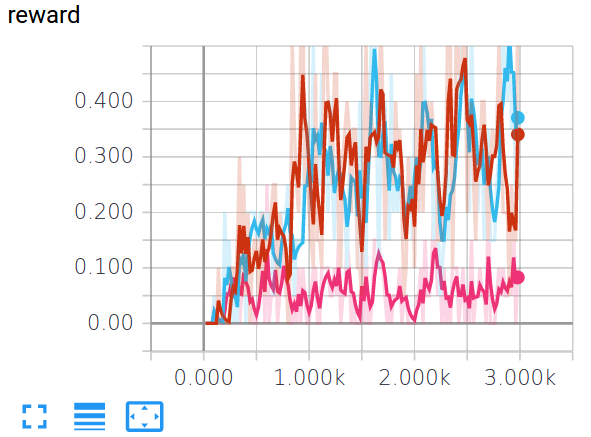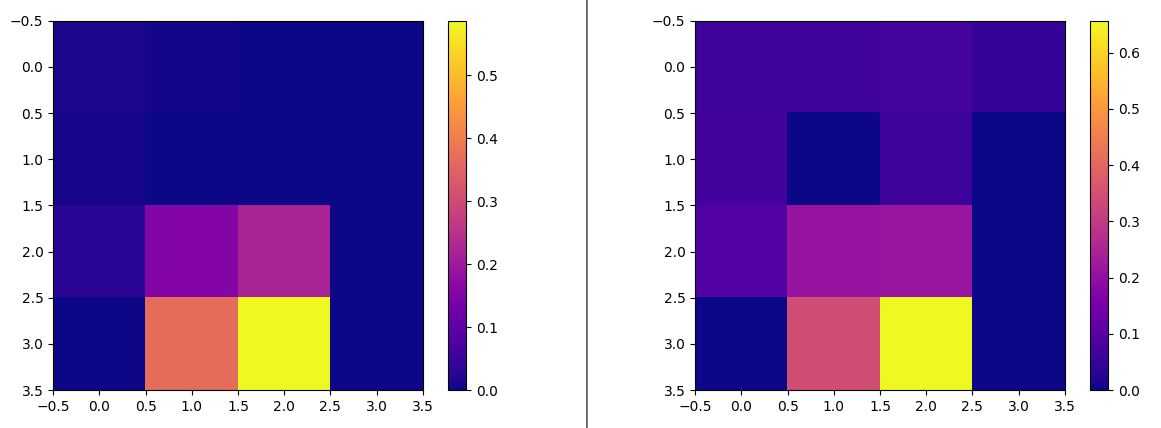Back to basics. I found that I didn’t write anything about reinforcement learning (RL) since I started blogging, although my research area has been RL for a while. I’m loving GANs too much these days :)

Same as the previous post, this is a series of my “reinventing-the-wheels” project to understand things well. Of course it depends on your available time, it always has some benefits to implement something by yourself. You may find how much effect a factor in an equation you didn’t care enough has, you may come up with something new as an extension of the study while you’re pondering about, or just simply you’ll get solid programming skill.

In this post, I’ll quickly review a tabular Q-learning and $\epsilon$-greedy action selection. Also I’ll list advanced topics, in particular, related to intrinsic reward to encourage the agent’s exploration.

Algorithms are implemented in reinventing-the-wheels/ML/rl/tabular_q.py

### The problem

Solve FrozenLake-v0 environment as an initial target. (More advanced version is also available FrozenLake8x8-v0)

• The agent controls the movement of a character in a grid world
• Some tiles of the grid are walkable, and others lead to the agent falling into the water
• The movement direction of the agent is uncertain
• The action consequences can be stochastic (because the ice is slippery and the agent moves towards unintended direction sometimes! This makes the problem much more difficult contrast to its simple setup)
• The agent is rewarded for finding a walkable path to a goal tile
• You receive a reward of 1 if you reach the goal, and zero otherwise
• The episode ends when you reach the goal or fall in a hole

The surface is described using a grid like the following

    SFFF     S : starting point, safe
FHFH     F : frozen surface, safe
FFFH     H : hole, fall to your doom
HFFG     G : goal, where the frisbee is located


### Tabular Q-learning

Since the number of states of the FrozenLake environment is limited, it’s possible to represent the environment as a matrix. The agent learns the value of each state based on Q-learning algorithm (I’ll not write every detail of the algorithm itself in this post).

To choose an action, the agent needs to estimate the value of each state and keep updating the estimates based on experiences. The simplest form of updating rule is as follows:

This simply says “update your old estimate based on the error weighted by step size”

The code looks like this (although it can be written in a single line, I implemented it step by step as follows).

At the first line of the code, you calculate the sum of the reward you just got from your step and the next state’s value. If your estimate is precise, this quantity should be the same with the estimate of the state’s value of your previous state (Bellman equation) so the difference is the error as we saw at the second line. OK, now the learning rule itself is clear. Next step!

### $\epsilon$-greedy

One of the well-known key challenges in RL is the “explore-exploit” tradeoff. For instance, your agent knows to step forward in a particular state $s$ will be rewarded but quite not sure about the right pathway growing from the state $s$. Well, the agent can just exploit its knowledge and enjoy known reward, but what if the right path leads the agent to more rewarding states? So, it’s better to balance the exploration and exploitation well. One simple technique to encourage the agent to explore is “$\epsilon$-greedy” algorithm.

#### 1-1. Simple $\epsilon$-greedy

This is a sample implementation of a simple $\epsilon$-greedy algorithm. First, you draw a random number and compare it with a pre-specified variable eps. Exploit if the drawn random number is greater than eps, or exploit.

#### 1-2. Decaying $\epsilon$-greedy

Another variation of implementing $\epsilon$-greedy is ‘decaying’ $\epsilon$-greedy. The previous implementation pre-specified the value of eps and it’s fixed throughout the iteration. It’s reasonable, however, to explore more at the early stage of the learning process and gradually increase the ratio of exploitation towards the end of the learning.

To make our agent behave like this, let’s use decaying eps with the following equation:

This controls the value of eps as follows and the eps gradually go down (which means less exploration as training goes). This makes sense because the agent needs to explore more in the early stage of its learning process as the agent doesn’t know much about the environment and needs to collect many different action/observation patterns. Eventually, however, optimal behavior is gonna be known based on try-and-errors and less exploration is needed then. It’s time to mostly exploit acquired knowledge.With this, code looks like this.

This $\epsilon$-greedy style exploration is used in a DeepMind’s sensational paper in which they showed that their Deep Q-learning agent can achieve a super-human level of playing Atari games.

The problem I personally think is that the hyperparameters, more specifically, eps_decay, for decay scheduling is a bit annoying and would like to avoid this tuning if possible.

#### Experiments

The figure below is a comparison of a simple greedy agent (pink), $\epsilon$-greedy agent (red), and $\epsilon$-greedy with decay (blue).

As reward is 0 or 1 in FrozenLake and is messy to see a performance, the values are average of every 20 epochs. You can see that $\epsilon$-greedy (with/without decaying) outperforms a simple greedy strategy. #As stated previously, the environment has stochasticity because of its slippery floor. If you set is_slippery=False to this environment, the problem is much much easier and the agent’s performance shows a sharp increaseOne more study here. The heatmap below is Q values after training for each greedy (left) and $epsilon$-greedy (right). The right figure indicates that this agent visited more cells than the left one as some upper cells have higher values.## Going further

#### Simple extensions: Adding noise to weights or observation

Some researches are showing that a simple idea of adding noises to a neural network’s parameters works well to encourage exploration.

#### Uncertainty major

Although $\epsilon$-greedy action selection makes an agent explore non-greedy actions, there is no notion about “how good that non-greedy action to take is among others”. Upper Confidence Bound (UCB) was introduced to think about uncertainties of estimates.

$N_t(a)$ denotes the number of times that action $a$ has been selected prior to time $t$ and this square root term works as an uncertainty measure. The intuition is that if you’ve already taken action $a$ many times in the past, you’re pretty certain about that action’s consequence. In the square root term above, the more $a$ is taken, the less the resulting quantity will be (more certain). The numerator is a logarithm so it grows relatively slowly.

#### Intrinsic reward, curiosity, surprise

Exploration is really an interesting problem, in particular, if an agent rarely finds/gets rewards (sparse reward problem), it’s super hard to determine what to do in that situation. We saw that in Q-learning you need to estimate the value of the states so that you can pick a possibly rewarding trajectory but this is simply impossible since there is no clue to update your estimates. In this case, the so-called “intrinsic reward” (or “Curiosity”) can help.

The basic strategy is to encourage an agent to visit unexplored states by adding additional rewards. Curiosity is a rhetorical naming for this because the agent can get rewarded by satisfying its curiosity by experiencing something new.

A Basic concept itself is intuitive and easy to understand. An agent will get rewarded by satisfying its internal curiosity by knowing the environment further (e.g. visit the unexplored area of the maze).

Although this post only covers the basic strategy of exploration, there are many interesting ideas related to this topic. I have a github repository to keep my paper summary and there are some information related to the topics above so check it if you are interested in. Also I’m implementing something related to this topic and will write a blog in the near future.

Whenever I take a breath and naively look at what we are struggling to reverse engineer, I feel humble and am amazed that how children inherently start exploring and we naturally balance and dynamically adjust exploration and exploitation in our life. So many things are still waiting to be discovered. Keep exploring!